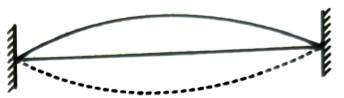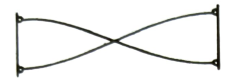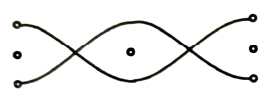Stationary waves
Question

# String of length L whose one end is x = 0 vibrates according to the relations given in column-I. Select matching entries from column-lI including nodes or antinodes at the end points of string.Column-IColumn-II(a)$\mathrm{y}=\mathrm{Asin}\frac{\mathrm{\pi x}}{\mathrm{L}}\mathrm{sin}\mathrm{\omega t}$(p)1 antinode, 2 nodes(b)$\mathrm{y}=\mathrm{Acos}\frac{\mathrm{\pi x}}{\mathrm{L}}\mathrm{sin}\mathrm{\omega t}$(q)3 nodes, 2 antinodes(c)$\mathrm{y}=\mathrm{Asin}\frac{2\mathrm{\pi x}}{\mathrm{L}}\mathrm{sin}\mathrm{\omega t}$(r)2 antinodes, 1 node(d)$\mathrm{y}=\mathrm{Acos}\frac{2\mathrm{\pi x}}{\mathrm{L}}\mathrm{sin}\mathrm{\omega t}$(s)3 antinodes, 2 nodes

Moderate
Solution

## (a) $\mathrm{K}=\frac{2\mathrm{\pi }}{\mathrm{\lambda }}=\frac{\mathrm{\pi }}{\mathrm{L}}⇒\frac{\mathrm{\lambda }}{2}=\mathrm{L}$(b)(c) $\mathrm{K}=\frac{2\mathrm{\pi }}{\mathrm{\lambda }}=\frac{2\mathrm{\pi }}{\mathrm{L}}⇒\mathrm{\lambda }=\mathrm{L}$(d)Get Instant Solutions